Pointwise product of functions

(diff) ← Older revision | Latest revision (diff) | Newer revision → (diff)

Definition

For two functions

Suppose$f$ and$g$ are functions. The pointwise product (often simply called the product) of the functions, denoted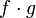$f \cdot g$, or sometimes simply as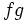$fg$, is defined as the function:$\! x \mapsto f(x)g(x)$

In other words, every element is sent to the product (in the sense of multiplication) of the values of$f$ and$g$ at that element.

The domain of the pointwise product of$f$ and$g$ is defined as the intersection of the domain of$f$ and the domain of$g$. This is necessary, because, for a pointwise product to make sense, both functions must be defined at the point.

For multiple functions

Suppose$f_1, f_2, \dots, f_n$ are functions. The pointwise product of these, denoted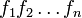$f_1f_2 \dots f_n$ or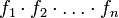$f_1 \cdot f_2 \cdot \dots \cdot f_n$, is defined as the function: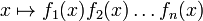$\! x \mapsto f_1(x)f_2(x) \dots f_n(x)$

In other words, every element is sent to the product (in the sense of multiplication) of the values of$f_1, f_2, \dots, f_n$ at that element.

The domain of the pointwise product of a bunch of functions is defined as the intersection of the domains of all the functions.

Relation with various operations

For two functions

Below we discuss how to perform various operations on the pointwise product$f \cdot g$ of$f$ and$g$, given knowledge of how to perform the operations on$f$ and$g$ individually.

Operation Verbal description How it's done
Graph$f \cdot g$ We are given the graphs of f and g (without necessarily having algebraic, numerical, or verbal descriptions of the functions) and we need a geometric method to sketch the graph of$f \cdot g$ too tricky?
Obtain explicit expression for$f \cdot g$ We are given algebraic expressions for$f$ and$g$ and need an explicit algebraic expression for$f \cdot g$. Part of a general procedure: finding pointwise combinations of functions by plugging in expressions. See also the piecewise case: finding pointwise combinations of piecewise functions by plugging in expressions.
Find limit of$f \cdot g$ at a point We know how to find limits of$f,g$ at points. limit of products is product of limits
Differentiate$f \cdot g$. We know how to differentiate$f$ and$g$ individually, we need to differentiate$f \cdot g$. product rule for differentiation:$(f \cdot g)' = (f' \cdot g) + (f \cdot g')$.
Integrate$f \cdot g$. We want to integrate$f \cdot g$ in terms of integration of simpler functions. We can try integration by u-substitution or integration by parts.